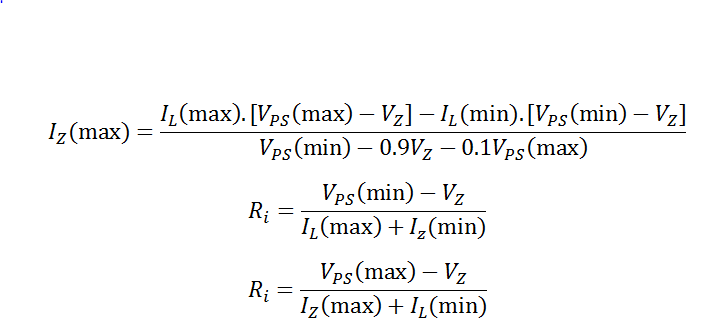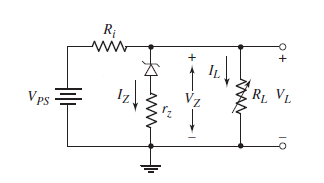# Zener Diode circuit design Calculator

This CalcTown calculator calculates the Input Resistance (Ri) in a Zener Diode voltage regulator circuit so that the designed specifications of input voltage and load resistance can be met.

*Please note that it has been assumed that the minimum Zener Current is 10% of the maximum Zener Current. Although in more practical applications, it is usually 20-30%.

**Out of the two resistance values shown in the output, please choose the one with lower magnitude and neglect the higher value.

V
V
mA
mA
V

#### Result

mA
K-Ohm
K-Ohmwhere

VPS = Voltage of the Power Source

IL = Current Flowing through the Load Resistance

IZ = Current Flowing through the Zener Diode

VZ = Zener Voltage

IZ(max)= Maximum Zener Current

and Load Resistance and Power Source Voltage are variable over a certain range.